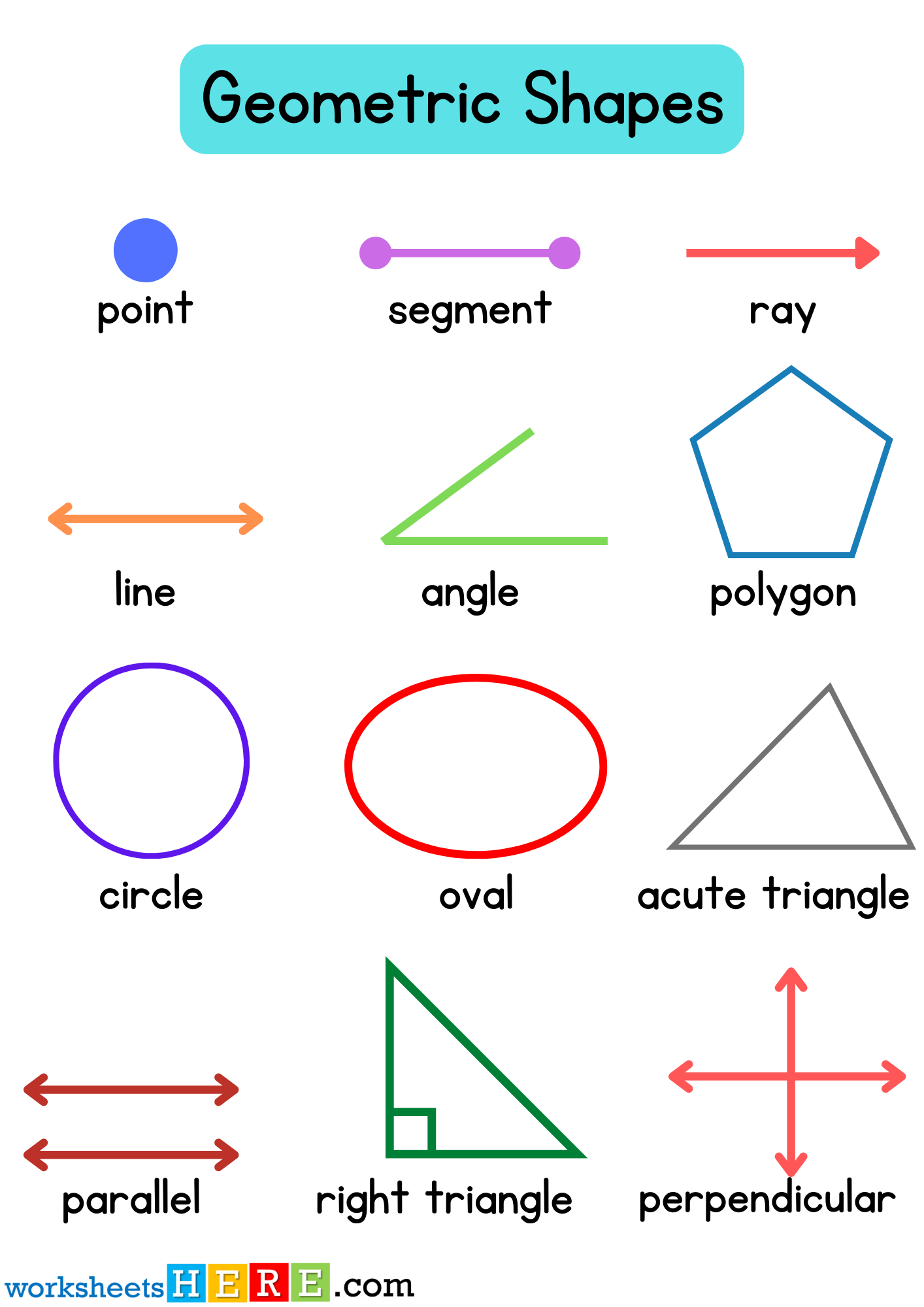# Geometric Shapes and Names PDF Worksheet For Kids

Geometric Shapes and Names PDF Worksheet For KidsFor Download PDF Worksheet, Click Here; Geometric Shapes and Names PDF Worksheet For Kids

##### Geometric Shapes List
• Nonagon
• Ellipse
• Rectangular
• Rectangular Prism
• Octagon
• Cone
• Triangle
• Scalene triangle
• Arrow
• Cube
• Cylinder
• Star
• Heptagon
• Hexagon
• Crescent
• Pyramid
• Right triangle
• Parallelogram
• Rhombus
• Square
• Pentagon
• Trapezoid
• Circle
• Oval
• Decagon
• Heart
• Cross

Geometric shapes can be named as figure or area closed by a boundary which is created by combining the specific amount of curves, points, and lines. There are different geometric shapes are Triangle, Circle, Square, etc. It is very important that you acquire the necessary understanding of the geometric shapes. For example; the common shapes in geometry like are square, rectangle, circle, and triangle. Here are some of geometric shapes and definition;

##### Square

A square is a four-sided area which is created by connecting 4 line segments. The line segments in the square are all of the equal lines. They come together to form 4 right angles.

##### Circle

If we talk about a circle which is another shape of geometry has no straight lines. It is rather a combination of curves that are all linked. In a circle, there is no angle to be found.

##### Rectangle

Similar to a square, a rectangle is also created by linking four line segments. However, there is only a difference between a square and a rectangle. In addition a rectangle, has two line segments which are longer than the other two line segments. So, in geometry, a rectangle is also called as an elongated square.

##### Triangle

Triangle comprises three linked line segments. Unlike, a square, in a triangle, the angles can be of distinct measurements. They aren’t always the right angles. Triangles are called, depending upon the type of angles which is found within the triangle itself. For example, if a triangle has one right angle, it will be known as a right-angled triangle.